# Introduction

The `adept` package implements ADaptive Empirical Pattern Transformation (ADEPT) method1 for pattern segmentation from a time-series. ADEPT is optimized to perform fast, accurate walking strides segmentation from high-density data collected with a wearable accelerometer during walking. The method was validated using data collected with sensors worn at left wrist, left hip and both ankles.

This vignette introduces ADEPT algorithm and demonstrates the usage of `segmentPattern` function which implements ADEPT approach. Here, we focus on examples with simulated data; see the Walking strides segmentation with adept2 for the example of walking stride segmentation in real-life data.

ADEPT identifies patterns in a time-series `x` via maximization of chosen similarity statistic (correlation, covariance, etc.) between a time-series `x` and a pattern template(s). It accounts for variability in both (1) pattern duration and (2) pattern shape.

## Pattern template

We define a pattern template as a 1-dimensional numeric vector which values represent the pattern of interest (e.g. accelerometry data of a human stride).

• See  where we show a way to derive walking stride templates from accelerometry data collected at left wrist, left hip and both ankles.
• See  where we employed templates derived in  to segment stride pattern from another accelerometry data set.

In this vignette, a pattern template is a simulated data vector.

# Install and load `adept` package

Install `adept` package from GitHub.

``````# install.packages("devtools")

Load `adept` and other packages.

``````library(adept)
library(magrittr)
library(ggplot2)``````

# Pattern segmentation with `adept` package

The examples below are organized into suites. Examples within one suite share data simulation settings, for example: Examples 1: signal with no noise, same-length pattern.

## Examples 1: signal with no noise, same-length pattern

### Simulate data

We simulate a time-series `x`. We assume that

• `x` is collected at a frequency of 100 Hz,
• there is one shape of pattern present within `x`,
• each pattern lasts 1 second,
• there is no noise in the collected data.
``````true.pattern <- cos(seq(0, 2 * pi, length.out = 100))
x <- c(true.pattern, replicate(10, true.pattern[-1]))

data.frame(x = seq(0, 1, length.out = 100), y = true.pattern) %>%
ggplot() + geom_line(aes(x = x, y = y), color = "red") +
theme_bw(base_size = 9) + labs(x = "Phase", y = "Value", title = "Pattern")``````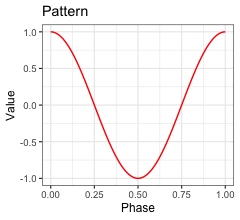``````data.frame(x = seq(0, by = 0.01, length.out = length(x)), y = x) %>%
ggplot() + geom_line(aes(x = x, y = y)) +  theme_bw(base_size = 9) +
labs(x = "Time [s]", y = "Value", title = "Time-series x")``````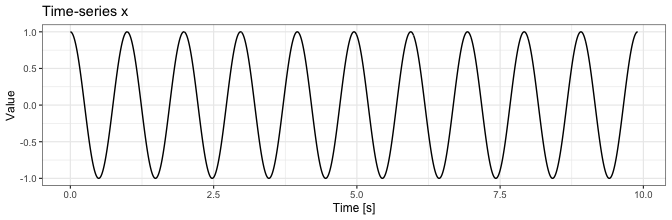### Example 1(a): segment pattern

We use `segmentPattern` to segment pattern from a time-series `x`. We assume that a perfect template is available. We use a grid of potential pattern durations of {0.9, 0.95, 1.03, 1.1} seconds; the grid is imperfect in a sense it does not contain the duration of the true pattern used in `x` simulation.

``````out <- segmentPattern(
x = x,
x.fs = 100,
template = true.pattern,
pattern.dur.seq = c(0.9, 0.95, 1.03, 1.1),
similarity.measure = "cor",
compute.template.idx = TRUE)
out
#>    tau_i T_i     sim_i template_i
#> 1      4  95 0.9987941          1
#> 2     98 103 0.9992482          1
#> 3    202  95 0.9987941          1
#> 4    296 103 0.9992482          1
#> 5    400  95 0.9987941          1
#> 6    494 103 0.9992482          1
#> 7    598  95 0.9987941          1
#> 8    692 103 0.9992482          1
#> 9    796  95 0.9987941          1
#> 10   895  95 0.9987941          1``````

#### `segmentPattern` output

The segmentation result is a data frame, where each row describes one identified pattern occurrence:

• `tau_i` - index of `x` where pattern starts,
• `T_i` - pattern duration, expressed in `x` vector length,
• `sim_i` - similarity between a template and `x`,
• `template_i` - index of a template best matched to a time-series `x` (here: one template was used, hence all `template_i`’s equal 1).

See `?segmentPattern` for details.

### Example 1(b): use `pattern.dur.seq` to modify a grid of pattern duration

We next generate a dense grid of potential pattern durations, including value `1.0` seconds used in the `x` simulation. A perfect match (`sim_i = 1`) between a time-series `x` and a template is obtained.

``````out <- segmentPattern(
x = x,
x.fs = 100,
template = true.pattern,
pattern.dur.seq = c(0.9, 0.95, 1, 1.03, 1.1),
similarity.measure = "cor",
compute.template.idx = TRUE)
out
#>    tau_i T_i sim_i template_i
#> 1      1 100     1          1
#> 2    100 100     1          1
#> 3    199 100     1          1
#> 4    298 100     1          1
#> 5    397 100     1          1
#> 6    496 100     1          1
#> 7    595 100     1          1
#> 8    694 100     1          1
#> 9    793 100     1          1
#> 10   892 100     1          1``````

### Example 1(c): use `x.fs` to modify `x` time-series frequency

We use `x.fs` to modify `x` time-series frequency, expressed in a number of observations in seconds, and we adjust `pattern.dur.seq` accordingly. We observe that results are the same as in Example 1(b).

``````out <- segmentPattern(
x = x,
x.fs = 10,   ## Assumed data frequency of 10 observations per second
template = true.pattern,
pattern.dur.seq = c(0.9, 0.95, 1, 1.03, 1.1) * 10, ## Adjusted accordingly
similarity.measure = "cor",
compute.template.idx = TRUE)
out
#>    tau_i T_i sim_i template_i
#> 1      1 100     1          1
#> 2    100 100     1          1
#> 3    199 100     1          1
#> 4    298 100     1          1
#> 5    397 100     1          1
#> 6    496 100     1          1
#> 7    595 100     1          1
#> 8    694 100     1          1
#> 9    793 100     1          1
#> 10   892 100     1          1``````

## Examples 2: signal with no noise, pattern duration varies

### Simulate data

We simulate a time-series `x`. We assume that

• `x` is collected at a frequency of 100 Hz,
• there is one shape of a pattern present within `x`,
• patterns have various duration,
• there is no noise in the collected data.
``````set.seed(1)
true.pattern <- cos(seq(0, 2 * pi, length.out = 200))
x <- numeric()
for (vl in seq(70, 130, by = 10)){
true.pattern.s <- approx(
seq(0, 1, length.out = 200),
true.pattern, xout = seq(0, 1, length.out = vl))\$y
x <- c(x, true.pattern.s[-1])
if (vl == 70) x <- c(true.pattern.s, x)
}

data.frame(x = seq(0, by = 0.01, length.out = length(x)), y = x) %>%
ggplot() + geom_line(aes(x = x, y = y)) +  theme_bw(base_size = 9) +
labs(x = "Time [s]", y = "Value", title = "Time-series x")``````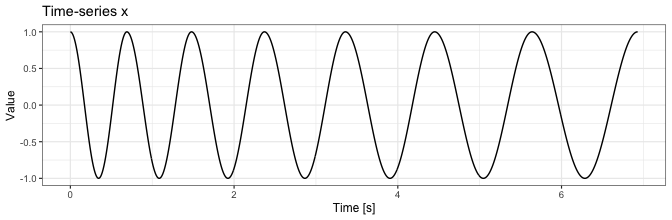``````
## Function to plot segmentation results with ggplot2
library(ggplot2)
out.plot1 <- function(val, out, fs = 100){
yrange <- c(-1, 1) * max(abs(val))
y.h <- 0
plt <- ggplot()
for (i in 1:nrow(out)){
tau1_i <- out[i, "tau_i"]
tau2_i <- tau1_i + out[i, "T_i"] - 1
tau1_i <- tau1_i/fs
tau2_i <- tau2_i/fs
plt <-
plt +
geom_vline(xintercept = tau1_i, color = "red") +
geom_vline(xintercept = tau2_i, color = "red") +
annotate(
"rect",
fill = "pink",
alpha = 0.3,
xmin = tau1_i,
xmax = tau2_i,
ymin = yrange,
ymax = yrange
)
}
geom_line.df <- data.frame(x = seq(0, by = 1/fs, length.out = length(val)), y = val)
plt <-
plt +
geom_line(data = geom_line.df,
aes(x = x, y = y),
color = "black",
size = 0.3) +
theme_bw(base_size = 9) +
labs(x = "Time [s]", y = "Black line: x",
title = "Black line: signal x\nRed vertical lines: start and end points of identified pattern occurrence\nRed shaded area: area corresponding to identified pattern occurrence")
plot(plt)
}``````

### Example 2(a): segment pattern

We use a dense grid of potential pattern duration, including all values used in the `x` simulation to again obtain the perfect match (`sim_i = 1`). In this example, the start and the end points of identified patterns are connected (see figure below).

``````out <- segmentPattern(
x = x,
x.fs = 100,
template = true.pattern,
pattern.dur.seq = 60:130 * 0.01,
similarity.measure = "cor",
compute.template.idx = TRUE)
out
#>   tau_i T_i sim_i template_i
#> 1     1  70     1          1
#> 2    70  80     1          1
#> 3   149  90     1          1
#> 4   238 100     1          1
#> 5   337 110     1          1
#> 6   446 120     1          1
#> 7   565 130     1          1

out.plot1(x, out)``````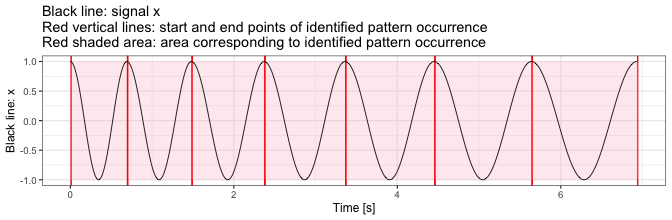### Example 2(b): use `pattern.dur.seq` to modify a grid of pattern duration

Next, we use a less dense grid of potential pattern duration. We observe that perfect match (`sim_i = 1`) between a template and time-series `x` is no longer obtained. Note:

• A less dense `pattern.dur.seq` grid yields a shorter time of method execution.
• As explained later, when peak detection tuning is used, having a “maximally dense” pattern duration grid does not contribute much.
``````out <- segmentPattern(
x = x,
x.fs = 100,
template = true.pattern,
pattern.dur.seq = c(0.6, 0.9, 1.2),
similarity.measure = "cor",
compute.template.idx = TRUE)
out
#>   tau_i T_i     sim_i template_i
#> 1     6  60 0.9954038          1
#> 2    80  60 0.9887682          1
#> 3   149  90 1.0000000          1
#> 4   243  90 0.9976255          1
#> 5   347  90 0.9934059          1
#> 6   446 120 1.0000000          1
#> 7   570 120 0.9985549          1

out.plot1(x, out)``````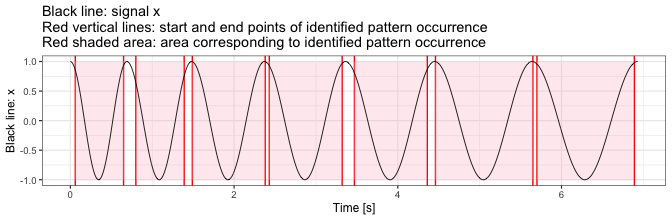### Example 2(c): use `similarity.measure` to modify similarity statistic

We use `similarity.measure` to modify the similarity statistic. We observe that `sim_i` values in the result data frame change and the segmentation results change slightly too. The explanation is that a change of similarity statistic takes an effect on ADEPT iterative maximization procedure.

``````out <- segmentPattern(
x = x,
x.fs = 100,
template = true.pattern,
pattern.dur.seq = c(0.6, 0.9, 1.2),
similarity.measure = "cov",  ## Use covariance as a similarity statistic
compute.template.idx = TRUE)
out
#>   tau_i T_i     sim_i template_i
#> 1     6  60 0.6440590          1
#> 2    65  90 0.7338618          1
#> 3   154  90 0.6711920          1
#> 4   243  90 0.6675955          1
#> 5   332 120 0.7320470          1
#> 6   451 120 0.6883499          1
#> 7   570 120 0.6786581          1

out.plot1(x, out)``````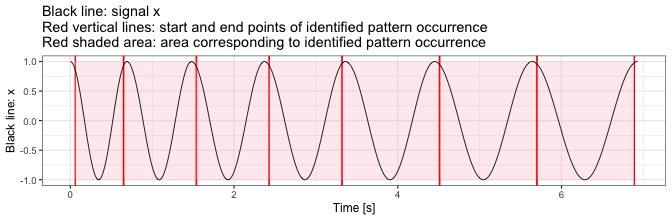## Examples 3: signal with no noise, pattern duration and pattern shape vary

### Simulate data

We simulate a time-series `x`. We assume that

• `x` is collected at a frequency of 100 Hz,
• there are two shapes of pattern present within `x`,
• patterns have various duration,
• there is no noise in the collected data.
``````true.pattern.1 <- cos(seq(0, 2 * pi, length.out = 200))
true.pattern.2 <- true.pattern.1
true.pattern.2[70:130] <- 2 * true.pattern.2[min(70:130)] + abs(true.pattern.2[70:130])
x <- numeric()
for (vl in seq(70, 130, by = 10)){
true.pattern.1.s <- approx(
seq(0, 1, length.out = 200),
true.pattern.1, xout = seq(0, 1, length.out = vl))\$y
true.pattern.2.s <- approx(
seq(0, 1, length.out = 200),
true.pattern.2, xout = seq(0, 1, length.out = vl))\$y
x <- c(x, true.pattern.1.s[-1], true.pattern.2.s[-1])
if (vl == 70) x <- c(true.pattern.1.s, x)
}

data.frame(x = seq(0, by = 0.01, length.out = length(x)), y = x) %>%
ggplot() + geom_line(aes(x = x, y = y)) +  theme_bw(base_size = 9) +
labs(x = "Time [s]", y = "Value", title = "Time-series x")``````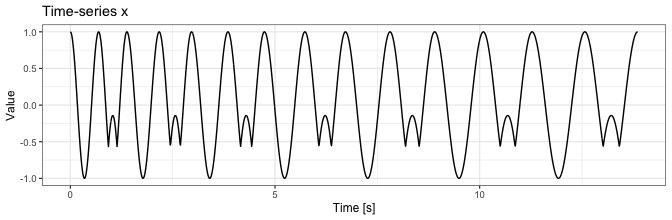``````plt1 <-
data.frame(x = seq(0, 1, length.out = length(true.pattern.1)), y = true.pattern.1) %>%
ggplot() + geom_line(aes(x = x, y = y), color = "red") +
theme_bw(base_size = 9) + labs(x = "Phase", y = "Value", title = "Pattern 1") +
scale_y_continuous(limits = c(-1,1))
plt2 <-
data.frame(x = seq(0, 1, length.out = length(true.pattern.2)), y = true.pattern.2) %>%
ggplot() + geom_line(aes(x = x, y = y), color = "red") +
theme_bw(base_size = 9) + labs(x = "Phase", y = "Value", title = "Pattern 2") +
scale_y_continuous(limits = c(-1,1))
plt1;plt2``````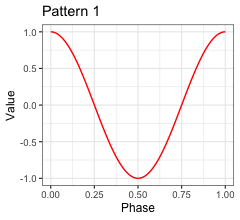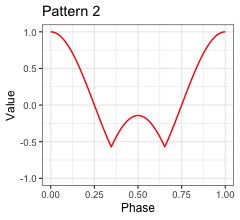### Example 3(a): segment pattern

To segment pattern from `x`, we use a dense grid of potential pattern duration. We use a `template` object consisting of one of the two true patterns used in `x` simulation.

``````out <- segmentPattern(
x = x,
x.fs = 100,
template = true.pattern.1, ## Template consisting of one out of two true patterns
pattern.dur.seq = 60:130 * 0.01,
similarity.measure = "cor",
compute.template.idx = TRUE)
out
#>    tau_i T_i     sim_i template_i
#> 1      1  70 1.0000000          1
#> 2     70  70 0.9290889          1
#> 3    139  80 1.0000000          1
#> 4    218  80 0.9291321          1
#> 5    297  90 1.0000000          1
#> 6    386  90 0.9287727          1
#> 7    475 100 1.0000000          1
#> 8    574 100 0.9287316          1
#> 9    673 110 1.0000000          1
#> 10   782 110 0.9285349          1
#> 11   891 120 1.0000000          1
#> 12  1010 120 0.9284384          1
#> 13  1129 130 1.0000000          1
#> 14  1258 130 0.9283518          1

out.plot1(x, out)``````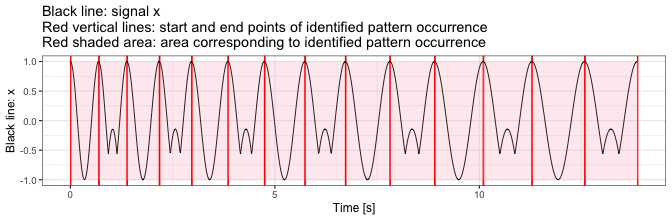### Example 3(b): use `similarity.measure.thresh` to modify the threshold of minimal similarity

We use a similarity threshold to segment only those patterns for which similarity (here: correlation) is greater than 0.95. Note that using the threshold may substantially speed up method execution when working with a large data set.

``````out <- segmentPattern(
x = x,
x.fs = 100,
template = true.pattern.1,
pattern.dur.seq = 60:130 * 0.01,
similarity.measure = "cor",
similarity.measure.thresh = 0.95,
compute.template.idx = TRUE)
out
#>   tau_i T_i sim_i template_i
#> 1     1  70     1          1
#> 2   139  80     1          1
#> 3   297  90     1          1
#> 4   475 100     1          1
#> 5   673 110     1          1
#> 6   891 120     1          1
#> 7  1129 130     1          1

out.plot1(x, out)``````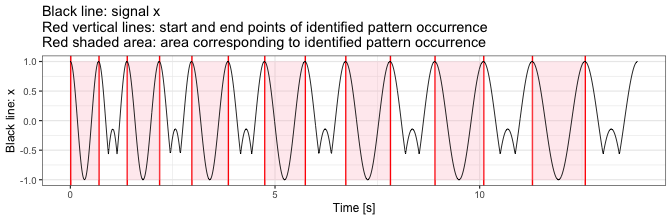### Example 3(c): use multiple templates simultaneously

We next use a `template` object consisting of both true patterns used in `x` simulation. We observe that the index of a pattern template best matched to a pattern in the time-series `x` is `1` and `2` interchangeably.

``````out <- segmentPattern(
x = x,
x.fs = 100,
template = list(true.pattern.1, true.pattern.2),
pattern.dur.seq = 60:130 * 0.01,
similarity.measure = "cor",
compute.template.idx = TRUE)
out
#>    tau_i T_i sim_i template_i
#> 1      1  70     1          1
#> 2     70  70     1          2
#> 3    139  80     1          1
#> 4    218  80     1          2
#> 5    297  90     1          1
#> 6    386  90     1          2
#> 7    475 100     1          1
#> 8    574 100     1          2
#> 9    673 110     1          1
#> 10   782 110     1          2
#> 11   891 120     1          1
#> 12  1010 120     1          2
#> 13  1129 130     1          1
#> 14  1258 130     1          2

out.plot1(x, out)``````## Examples 4: signal with noise, pattern duration and pattern shape vary

### Simulate data

We simulate a time-series `x`. We assume that

• `x` is collected at a frequency of 100 Hz,
• there are two shapes of a pattern present within `x`,
• patterns have various duration,
• there is noise in the collected data.
``````## Generate `x` as a noisy version of a time-series generated in *Examples 3*.
set.seed(1)
x <- x + rnorm(length(x), sd = 0.5)

data.frame(x = seq(0, by = 0.01, length.out = length(x)), y = x) %>%
ggplot() + geom_line(aes(x = x, y = y), size = 0.3) +  theme_bw(base_size = 9) +
labs(x = "Time [s]", y = "Value", title = "Time-series x")``````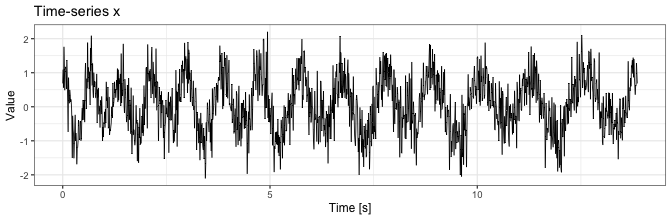### Example 4(a): segment pattern

We use two templates simultaneously in segmentation.

``````out <- segmentPattern(
x = x,
x.fs = 100,
template = list(true.pattern.1, true.pattern.2),
pattern.dur.seq =  60:130 * 0.01,
similarity.measure = "cor",
compute.template.idx = TRUE)
out
#>    tau_i T_i     sim_i template_i
#> 1      4  63 0.8615665          1
#> 2     76  62 0.7351057          2
#> 3    137  84 0.7708233          1
#> 4    220  76 0.6768561          2
#> 5    295  92 0.8507212          1
#> 6    391  83 0.7404364          2
#> 7    473 106 0.8300020          1
#> 8    582  87 0.6515298          2
#> 9    668 118 0.8047385          1
#> 10   785 106 0.6958617          2
#> 11   890 123 0.7927484          1
#> 12  1015 113 0.6789908          1
#> 13  1129 130 0.7938183          1
#> 14  1265 123 0.7686607          2

out.plot1(x, out)``````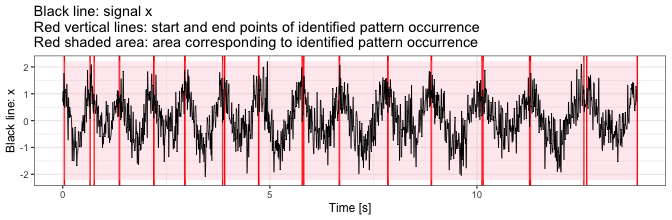### Example 4(b): use `x.adept.ma.W` to smooth `x` for similarity matrix computation

We use `x.adept.ma.W` to define a length of a smoothing window to smooth `x` for similarity matrix computation; `x.adept.ma.W` is expressed in seconds and the default is `NULL` (no smoothing applied).

Smoothing of a time-series `x`

Function `windowSmooth` allows observing the effect of smoothing for different values of smoothing window length `W`. `W` is expressed in seconds. Here, `W = 0.1` seconds seems to be a plausible choice.

``````x.smoothed <- windowSmooth(x = x, x.fs = 100, W = 0.1)

data.frame(x = seq(0, by = 0.01, length.out = length(x.smoothed)), y = x.smoothed) %>%
ggplot() + geom_line(aes(x = x, y = y)) +  theme_bw(base_size = 9) +
labs(x = "Time [s]", y = "Value", title = "Time-series x smoothed")
#> Warning: Removed 8 rows containing missing values (geom_path).``````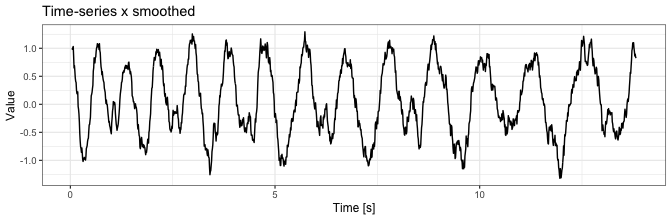Use `x.adept.ma.W = 0.1` and compare with results from Example 4(a). Observe that using a smoothed version of `x` in similarity matrix computation is pronounced in `sim_i` values in the output data frame, as well as in a slight change in `tau_i` and `T_i` values.

``````out <- segmentPattern(
x = x,
x.fs = 100,
template = list(true.pattern.1, true.pattern.2),
pattern.dur.seq =  60:130 * 0.01,
similarity.measure = "cor",
compute.template.idx = TRUE)
out
#>    tau_i T_i     sim_i template_i
#> 1      4  63 0.9931174          1
#> 2     75  63 0.9683646          2
#> 3    139  79 0.9683054          1
#> 4    217  80 0.9748040          2
#> 5    296  94 0.9802473          1
#> 6    391  82 0.9462213          2
#> 7    472 106 0.9855837          1
#> 8    578  93 0.9608881          2
#> 9    670 115 0.9887225          1
#> 10   784 107 0.9562694          2
#> 11   896 113 0.9734575          1
#> 12  1008 127 0.9703118          1
#> 13  1134 116 0.9606235          1
#> 14  1266 122 0.9593345          2

out.plot1(x, out)``````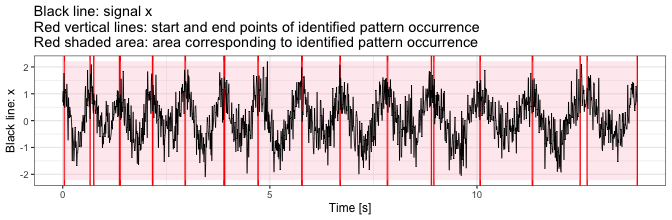### Example 4(c): use fine-tune procedure for peak detection

We employ a fine-tuning procedure for stride identification.

Fine-tune procedure `"maxima"`

Fine-tune procedure `"maxima"` tunes preliminarily identified start and end of a pattern occurrence so as they correspond to local maxima of `x` found within neighborhoods of the preliminary locations.

• The parameter `finetune.maxima.nbh.W`, expressed in seconds, defines a length of the two neighborhoods within which we search for local maxima.
• Smoothed version of `x` may be used for local maxima search (as presented later).
``````out <- segmentPattern(
x = x,
x.fs = 100,
template = list(true.pattern.1, true.pattern.2),
pattern.dur.seq =  60:130 * 0.01,
finetune = "maxima",
finetune.maxima.nbh.W = 0.3,
compute.template.idx = TRUE)
out
#>    tau_i T_i     sim_i template_i
#> 1      4  67 0.7183698          1
#> 2     70  78 0.4250660          2
#> 3    147  60 0.6161509          1
#> 4    206  98 0.5407526          2
#> 5    303  78 0.7570218          1
#> 6    380  83 0.3459633          2
#> 7    462 117 0.7566122          1
#> 8    578  93 0.5045821          2
#> 9    670 105 0.7153597          1
#> 10   774 113 0.5760463          2
#> 11   893 128 0.6794591          1
#> 12  1020 112 0.4026054          1
#> 13  1131 123 0.6736391          1
#> 14  1253 125 0.5570096          2

out.plot1(x, out)``````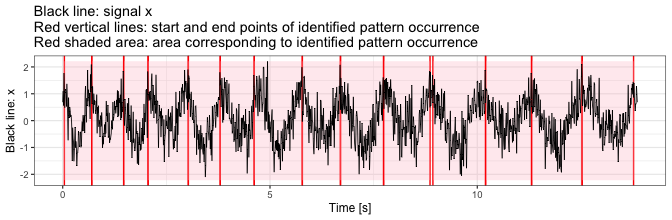We observe that almost all identified pattern occurrence start/end points are hitting the time point which our eyes identify as local `x` maxima.

### Example 4(d): use fine-tune procedure and smooth signal for peak detection

We smooth `x` for both similarity matrix computation (set `x.adept.ma.W = 0.1`) and for fine-tune peak detection procedure (set `finetune.maxima.nbh.W = 0.3`).

• Here, `W = 0.5` seconds seems to be a plausible choice for fine-tune peak detection procedure as it removes (“smooth together”) multiple local maxima of `x`, leaving out a single one.
``````x.smoothed.2 <- windowSmooth(x = x, x.fs = 100, W = 0.5)

data.frame(x = seq(0, by = 0.01, length.out = length(x.smoothed.2)), y = x.smoothed.2) %>%
ggplot() + geom_line(aes(x = x, y = y)) +  theme_bw(base_size = 9) +
labs(x = "Time [s]", y = "Value", title = "Time-series x smoothed aggresively")
#> Warning: Removed 48 rows containing missing values (geom_path).``````